# Using a DC/DC converter to power an ADC

In my previous blog, “Measuring PSR in an ADC”, I developed a complete PSR characterization procedure for a pipeline analog-to-digital converter (ADC) with low voltage differential signaling (LVDS) output and the methodology necessary to develop a power-supply specification for the maximum allowable ripple on the power-supply pins.

In this second installment of the blog, I will look at DC/DC converters both for the analog Vdd (AVDD) and the digital Vdd (DVDD) power supply.  Understanding how ADC power-supply pins react to DC/DC converters is critical, since DC/DC converters are part of most (if not all) powering schemes due to their high power efficiencies.

DC/DC converter efficiency can be in the mid-90 percent, providing the most power-efficient solution where power needs to be provided.  DC-DC converters have two main components to their internal power dissipation: static power dissipation and dynamic power dissipation

Static power dissipation corresponds to the energy needed to implement the functionality of the chip and is normally , where IQ is the quiescent current and VS the supply voltage.

Dynamic power dissipation corresponds to the energy needed to transmit the desired power to the load.  In DC-DC converters, the main contributors are energy lost:

• During the switching transition.
• Driving the output metal-oxide semiconductor field-effect transistor (MOSFET).
• In the MOSFET due to the internal series resistance.
• In the inductor used to average the output voltage resulting in the DC voltage.

Figure 1 shows the efficiency curve for DC-DC converters.

Figure 1: (a) TPS54120 DC/DC                                                                                                           (b) TPS62080 DC/DC

efficiency curve                                                                                                                      efficiency curve

Notice that the efficiency at the light load current drops dramatically and can indeed be worse than the efficiency of a linear regulator operating with a light load.  As such DC/DC converters are always better when loaded optimally or with a load varying within a specified range to ensure efficiency.  The highest efficiency for the TPS54120 is achieved above 500mA (>85 percent); for the TPS62080, it is from 300mA to 800mA (>90 percent).

I will not spend more time on the selection of DC/DC converters, as the best solution will depend on efficiency, cost, printed circuit board (PCB) area occupied versus available, possible electromagnetic interference, etc. …

For this analysis, I will use the TPS54120 and TPS62080 as example of the power-supply solution to evaluate the impact of different switching frequencies.  The TPS54120 is switching at 480kHz typical and the TPS62080 at 1.5MHz.  The TPS54120 is a dual-output device with one output being a low-noise, low-dropout linear regulator (LDO) and one output being a DC/DC converter.  The TPS62080 is a 1.2A high-efficiency step-down converter.

The TPS54120 is an extremely versatile device with a small PCB footprint that can be used as either a high-efficiency LDO (DC/DC and LDO used in series); see figure 2(a) or a power-tree optimizer (DC/DC and LDO used independently); see figure 2(b).

A) {12V to 1.8V at 1A; Efficiency ~ 70%}B) {2.3V to 1.2V at 3A; EfficiencyDC-DC ~ 83%}

{1.3V to 1.8V at 1A; EfficiencyLDO = 78.3%}

Figure 2: (a) High-efficiency LDO, (b) Power-tree optimizer configurations

Under no-load conditions, the time-domain response of the TPS54120 exhibits a 600µVPP ripple as shown in Figure 3.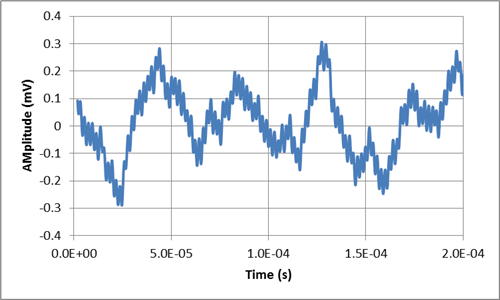Figure 3: TPS54120 DC/DC converter time-domain ripple

This time-domain ripple can be converted to a frequency-domain plot; see Figure 4.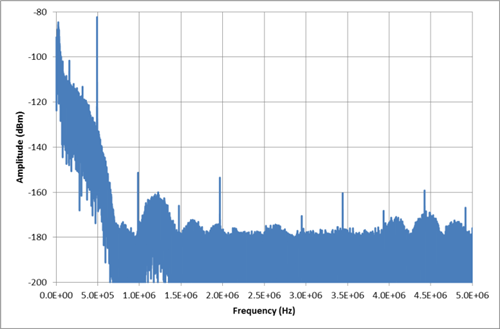Figure 4: TPS54120 DC/DC converter frequency-domain response

The TPS62080 is a 1.5MHz, 1.2A step down DC/DC converter.  Figure 5 illustrates its time-domain no-load response.  Note that this response exhibits an 8mVPP ripple.  This response is the TPS62080 snooze mode and will be similar to the TPS54120 DC/DC response once a load is attached to its output.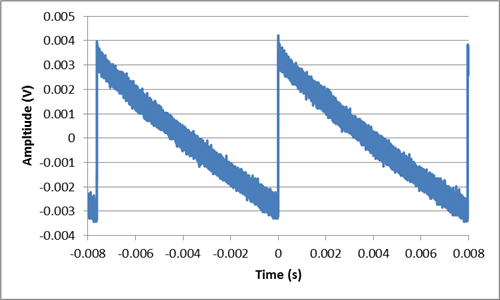Figure 5: TPS62080 time-domain no-load response

The fast Fourier transform (FFT) of Figure 5 is shown in Figure 6.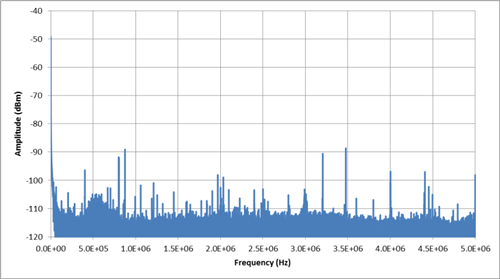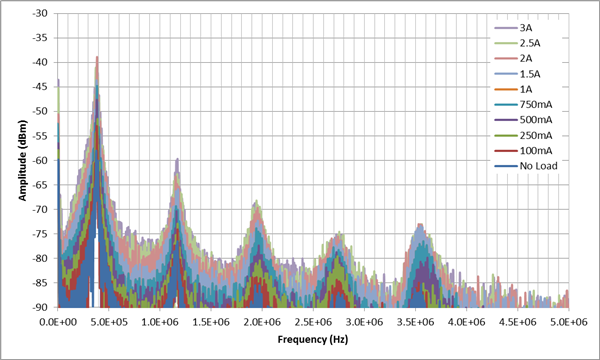Figure 7: TPS54120 FFT for various loads

I selected the TPS54120 and the TPS62080 due to their availability, allowing the evaluation of the ADC3444 with two very different switching frequencies (480kHz and 1.5MHz).

Looking first at the DVDD supply of the ADC3444 from the previous blogs, see “Measuring PSR in an ADC”, we know that the maximum allowable ripple at the DVDD pin is ~15mVPP.  Having a ripple below this value will ensure that not tone greater than -95dBFS will be present on the ADC FFT.

My evaluation is based on the block diagram shown in Figure 8.  In this figure, you can notice that the power supply source is a battery.  The use of a battery guarantees that the only source of power-supply switching-noise is originating in the DC/DC converter.  On the DVDD supply, under test, we inserted the DC/DC converter without any post-filtering, only maintaining the four 0.22µF capacitor at each ADC3444 DVDD pins.  The AVDD supply pin is powered of the same battery, but this time followed by a low-noise LDO, to ensure regulation at the appropriate voltage, but also provide an excellent rejection to any external switching noise and low thermal noise to the AVDD pins while providing the low impedance to the AVDD supply that is expected from a good power supply.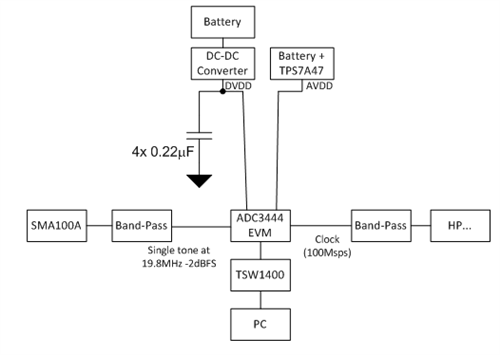Figure 8: ADC3444 DVDD test configuration

(Note: No ferrite bead was used as we are evaluating ADC3444 power-supply rejection ratio [PSRR] performance)

The ADC FFT results for both the TPS54120 DC-DC are shown in figure 9 below.

Figure 9: (a) ADC3444 reference plot; (b) ADC3444 FFT with TPS54120 as DVDD supply

We now have confirmation that our earlier calculation, from my “Measuring PSR in an ADC” post that a ripple below 600µVPP, the DVDD supply does not have any tone at the switching frequency above -95dBFS.  However, the DVDD supply also powers the internal clock circuitry, feeding the internal sample and hold circuit.  A convolution of the clock phase-noise with the switching frequency creates an energy spread between 200kHz all the way to 1MHz.

Even though the detail is not shown here, no tone was present around the signal at 19.8MHz as would be expected from the ADC3444 PSR curves.

Evaluating the same two DC/DC converters with the AVDD supply, (see block diagram figure 10), we have the results shown in figure 11.  In this case, we are connecting the Battery plus DC/DC arrangement to the AVDD supply and the Battery plus TPS7A47 to the DVDD supply.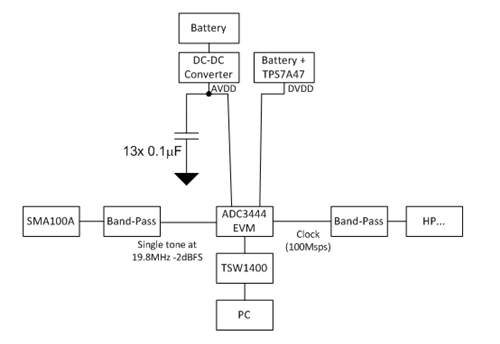Figure 10: AVDD evaluation block diagram

Knowing the DC/DC converter ripple voltage magnitude and frequency, this also confirms that the PSRR is not sufficient on the AVDD supply of the ADC.  Thus, we will have to decrease the switching-frequency amplitude of the DC/DC converter prior to connecting it to the ADC AVDD supply pin.

Figure 11: (a) and (c) ADC3444 reference plot; (b) ADC3444 DC-FFT with TPS54120 as AVDD supply;

(d) ADC3444 FFT with TPS54120 as AVDD supply (detail around single tone)

For the reference plot, the ADC3444 AVDD and DVDD supply pins are powered from the same 6V battery, but are using independent low noise LDOs.  (In this case, the TPS7A47).  Looking at the data, we can clearly see that a very low-level ripple, mostly due to using a 3A DC/-DC converter to drive a 40mA load, appears at the switching frequency and that there is no convolution here.  The up-converting of the switching frequency by the tone at 19.8MHz, however, is present; a -102dBFS signal clearly comes out of the noise floor.  Figure 12 summarizes the location of a sinewave noise spur.  The details are provided in the “Measuring PSR in an ADC” post.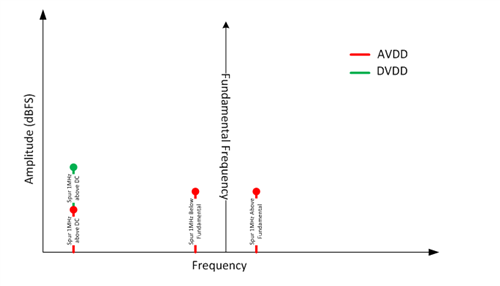Figure 12: Noise-spur locations

As the load in the DC/DC converter is going to be larger, the noise contribution of the power will increase, as seen in Figure 7, making it necessary to use a filtering strategy after the DC/DC converter.  Note that the typical noise increase is only characterized at room temperature and does not consider any other variations from the DC/DC converter, the external components or temperature..

So far in this series of blogs we have seen: how to measure the PSRR from an ADC, evaluated the ADC PSR over frequency and just developed the signal-chain degradation brought on by a poor power supply.  In the remainder blogs of this series, I will look at the power supply noise impact on the ADC performance and improvements from post filtering of the DC-DC converter, to finally develop a complete solution.